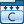## Generate Code from Rate-Based Model

Generate code from the rate-based model, not from the model test harness.

To generate code from a rate-based model:

1. In the Simulink® Toolstrip, on the Apps tab, click.

2. On the C Code tab that opens, click Build.

### Code Generation Report for Rate-Based Models

The code generation report for a rate-based model describes the timing of the model.

To open the code generation report, on the C Code tab, select .

Then, click Code Interface Report to view the timing of the functions in the generated code.### Generated Code Entry Points for Rate-Based Models

The combination of single-tasking versus multitasking and single-rate versus multirate controls the entry points in the generated code.

To enforce single-tasking, clear the Treat each discrete rate as a separate task configuration parameter. To allow for multitasking, select this parameter.

This table describes the generated code entry points for the `ex_rate_based_model` model, which contains two atomic subsystems named `Subsystem1` and `Subsystem2`.

• `Subsystem1` multiplies its input by 2.

• `Subsystem2` multiplies its input by 4.

ConfigurationExplicitly Scheduled RatesGenerated Code Entry Points

Single-rate.

`Subsystem1` and `Subsystem2` use a sample time of `0.2`.

One entry-point function called periodically every 0.2 seconds

```void ex_rate_based_model_step(void) { ex_rate_based_model_Y.Out1 = 2.0 * ex_rate_based_model_U.In1; ex_rate_based_model_Y.Out2 = 4.0 * ex_rate_based_model_U.In2; } ```

Multirate.

`Subsystem1` uses a sample time of `0.2`, and `Subsystem2` uses a sample time of `0.4`.

One entry-point function called periodically every 0.2 seconds.

In the entry-point function, a counter (`Timing.TaskCounters.TID`) and rate-scheduler function (`rate_scheduler`) determine which code runs at which sample times.

```void ex_rate_based_model_step(void) { ex_rate_based_model_Y.Out1 = 2.0 * ex_rate_based_model_U.In1; if (ex_rate_based_model_M->Timing.TaskCounters.TID == 0) { ex_rate_based_model_Y.Out2 = 4.0 * ex_rate_based_model_U.In2; } rate_scheduler(); } ```

The rate-scheduler function resets the counter when the entry-point function runs.

```static void rate_scheduler(void) { (ex_rate_based_model_M->Timing.TaskCounters.TID)++; if ((ex_rate_based_model_M->Timing.TaskCounters.TID) > 1) { ex_rate_based_model_M->Timing.TaskCounters.TID = 0; } }```

`Subsystem1` uses a sample time of `0.2`, and `Subsystem2` uses a sample time of `0.4`.
```void ex_rate_based_model_step0(void) { ex_rate_based_model_Y.Out1 = 2.0 * ex_rate_based_model_U.In1; } void ex_rate_based_model_step1(void) { ex_rate_based_model_Y.Out2 = 4.0 * ex_rate_based_model_U.In2; } ```